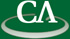Experimental Mathematics in the Normality of Pi

David Bailey, Computational Research Department, Lawrence Berkley National Laboratory

Thursday January 19th, 2006, in IRMACS 10900 at 3:30pm.

```
Modern computer technology now makes it possible to compute high-precision
numeric values of mathematical expressions and then to identify these values
analytically. This talk summarizes a number of recent results in this area,
including: (1) the 1996 discovery of a new formula for pi (with Peter Borwein
and Simon Plouffe), (2) the discovery of a large number of other BBP-like
identities, and (3) the recent discovery of analytic evaluations for some
integrals that arise in mathematical physics. Interestingly, there is a
connection between this theory and the age-old question of whether (and why)
the binary digits of constants such as pi and log(2) are "normal" (i.e.
statistically random in a certain sense). This realization has now led to a
simple proof (using ergodic theory techniques) of normality for a large
(uncountably infinite) class of real numbers.

```